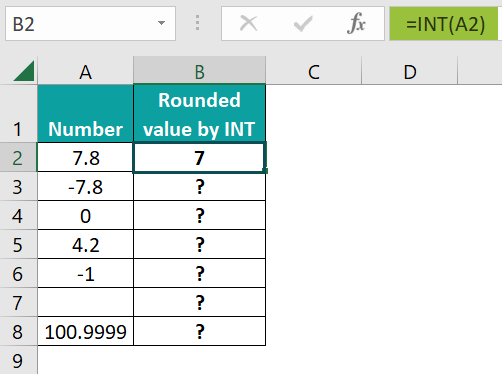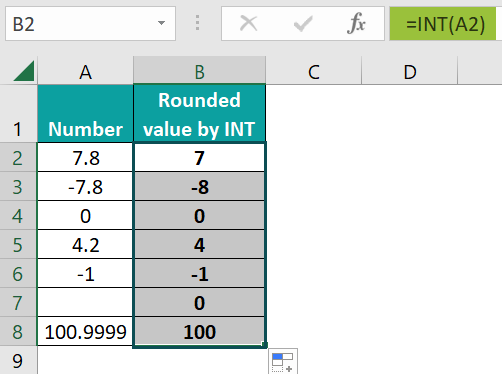# INT Excel FunctionArticle byExcelMojo Team## What is INT (Integer) Function in Excel?

The INT Excel function rounds a decimal number down to the nearest integer and returns it. It is one of the rounding functions in Excel which returns a positive or negative integer as an output. However, it returns the nearest lower integer in the case of positive numbers, and the negative numbers become more negative.

For example, below, we have two numbers. Let us apply the INT formula to them and check the output. Enter the formula =INT(A2) in cell B2. Press Enter and copy the formula to B3 as well. The INT function returns the integer 3 for the number 3.6. When we use the function on -3.6, it returns an integer away from zero, that is, -4.

###### Key Takeaways
• The INT Excel function rounds any number to the nearest lower integer in the case of positive numbers. It removes the decimal part of a number.
• In the case of negative numbers, the INT Excel function returns the nearest decimal number away from zero. Hence, the number becomes more negative. Using the MOD and INT excel functions, we can separate a number as an integer and decimal part.
• It is one of the rounding functions in Excel. The other rounding functions are TRUNC, ROUND, EVEN, etc.

### INT() Excel Formula

The syntax of the INT Excel function is as follows:

Arguments

Number (mandatory) – This is the number that you want to round to the nearest integer.

### How to Use INT(Integer) Excel Function?

The INT Excel function is one of the most used rounding functions. It is very similar to TRUNC Excel function. Now, we can use INT() in two ways. First, it can be entered manually or selected from the Formulas tab in the Excel ribbon.

#### Entering INT Excel Function Manually

Below is a list of numbers for which you must find the nearest integer value.

1. Place the cursor in cell B2 to find the nearest integer of the number 7.8.

2. Enter the formula as follows: =INT(A2) and press Enter.3. You get result 7 for argument 7.8. In the case of positive numbers, the function returns the lower nearest integer. Now, drag the Autofill handle from A2 to A8.As observed in the results, you can see that in the case of negative numbers like -7.8, the output is the nearest integer, which is away from zero, that is, -8.
Also, when the number is already an integer like -1(cell A6), the output is the same number.
For a blank cell like A7, the output obtained is zero.
Even if the number is 100.9999(cell A8), it is not rounded off to 101 but to 100.

#### Access from the Excel Ribbon

• Step 1: Choose an empty cell to get the output.
• Step 2: Go to the Formulas tab and click on the Math & Trig Drop Down arrow.
• Step 3: You get all the functions under this category. Select the INT function.
• Step 4: A pop-up window appears. Enter the “Number to get the nearest integer of that number.

Thus, the INT Excel function can be accessed in two ways, manually or through the Excel ribbon, as seen above.

### Examples

If you are left wondering about the use of the INT function in Excel, you can look at some examples below to understand its importance.

#### Example #1

Below is a set of different values. Let us apply the INT function to each number and check the outcome. Let us also compare it with a similar function in Excel, TRUNC. Like INT, the TRUNC Excel function removes the decimal part of a number and gives us just the integer part.

However, it is slightly different from the INT Excel function, which we will observe below.

• Step 1: Apply the function =INT(C4) in cell D4, to get the nearest integer for 156.54. Apply the function =TRUNC(C4) in cell E4.
• Step 2: You can observe that both the INT and TRUNC functions have given the same output, 156.

Here, TRUNC has removed the decimal part of 156.54, while INT has rounded the number to the nearest lower integer, 156. Drag the Autofill Excel handles in cells D4 and E4 up to cells D10 and E10, respectively.

Let us observe each result.

• 184.00 has been rounded off to 184 by both functions.
• INT has rounded off -254.5 to the nearest integer away from zero, -255, while the decimal part has been removed by TRUNC, thereby producing a different output, -254.
• Similarly, in the case of -2.9, INT gives the nearest integer away from zero, -3, while TRUNC removes the decimal and provides an output of -2.
• A non-numeric value gives an #VALUE! error for both functions.
• Both the INT and TRUNC functions supply a numeric value for the time value 12:00:00 AM. It is because Excel stores the time as a serial number. Therefore, you must format both the cells as Time in the Number group to obtain the time.

#### Example #2

In this INT Excel function example, we have date and time information in cells B2 to B8. Here, we must separate the time and date. The INT formula helps us separate the time and date with precision.

• Step 1: Excel stores Date as an integer, while Time is stored as a decimal fraction. To obtain only the date from Column B, you must get the integer part of the cell.
• Step 2: Enter =INT(B2) in cell C2. Press Enter. You get the integer part of cell B2, that is, the date.
• Step 3: Drag the Autofill handle up to cell C8. You get the date for all the cells.
• Step 4: You must get the time part alone from Column B. It’s simple! Just subtract the integer part obtained in Column C from the whole date and time in Column B.

Apply the formula =B2-C2 in cell D2 and press Enter.

• Step 5: Copy the formula from D2 to D8 by dragging the Autofill handle. You can find the time information in Column D. You can format Columns C and D as Date and Time, respectively, using the Numbers group of the Home tab.

#### Example #3

In this example, we have a list of employees who have served an organization. Their date of joining and date of leaving details have been provided. We must find the number of days each employee worked for the organization. We must also find the information on exact days and years’ count.

• Step 1: First, we must find the number of days the employee has worked. Since dates are stored as integers in Excel, you must subtract the joining date from the date of leaving.

Type the formula =INT(D3) – INT(C3) in cell E3. Press Enter. You get the number of days the first employee, Sam, worked for the organization.

• Step 2: Drag the handle from E3 to E13 to get the details on the number of days all the employees have worked for the organization.
• Step 3: To find the total duration the employees have worked in terms of years and days, you may use the functions INT and MOD. While INT gives you the integer part, which is the years’ count when you divide the total days by 365; MOD gives you the remainder, which represents the remaining days.

Apply the following function to cell F3 and press Enter.

=INT(E3/365)&” Years “&MOD(E3,365)&” Days”

Here,

• When you divide the days in Column E by 365, you get the number of years the employee has worked for the company.
• When you use MOD after dividing by 365, you get the days remaining after obtaining the years. MOD takes two arguments, MOD(number, divisor), and returns the remainder.
• ‘&’ is used to concatenate the different strings and operators.
• Step 4: Drag the handle from F3 to F13 to get the number of days and years each employee has worked.

Thus, the INT function returns the nearest integer for any value and can be used in various ways.

### Important Things to Note

• If the argument to the INT function is a text, we will get the #VALUE! Error in Microsoft Excel. However, if an integer is stored as a string, to convert string to INT excel function, you should use the VALUE Excel function.
• If the Excel cell reference supplied to the INT function is invalid, it will return a #REF! Error, and you will observe the INT excel function not working.
• INT() belongs to the class Math and Trig functions in Excel.
• For positive numbers, INT and TRUNC give the same output; however, INT returns an integer away from zero for negative numbers, while TRUNC removes the decimal part.

1. What does INT function do in Excel?

The INT Excel function returns the integer part of a decimal number. It also returns the nearest integer, lower than the number supplied. In the case of negative numbers, it returns the nearest integer away from zero. For instance, =INT(-3.5) gives an output of -4.

2. How do you format INT in Excel?

When any number is entered in an Excel cell, it is in the General format. However, if you convert it into the nearest integer using the INT Excel function, you can format it according to requirements, such as a number, date, etc., by right-clicking and choosing the Format Cells option. Then, select the Number tab in the pop-up window and format the INT function.

3. How do you round up to an INT in Excel?

If you have a decimal number, you can round it up to the nearest integer with several rounding functions available in Excel. Also, you can use the INT or TRUNC function to round a number to the nearest integer. The other rounding functions include ROUND, ODD, EVEN, and so on.